# On the ballooning instability of the coupled Alfvén and drift compressional modes

## Abstract

The paper examines the ballooning instability in gyrokinetic approximation taking into account the effects of finite-β, magnetic field line curvature, and diamagnetic drift. We used a simple model with a constant curvature of magnetic field lines which enabled us to obtain analytical results. The possible plasma oscillatory modes comprise the poloidal Alfvén and drift compressional modes, coupled due to the magnetic field line curvature and plasma inhomogeneity. The frequencies of these modes depend on the westward current value. As this value grows, the frequencies of these two branches approach to each other, and the branches are merged at some critical value of the current. Then an instability develops which is called the drift ballooning coupling instability. There are three major differences of the drift ballooning coupling instability from the ordinary MHD ballooning instability: (1) the drift ballooning coupling instability is not aperiodic, there is a real part of the oscillation frequency of the order of the drift frequency, (2) only the mode with the same direction of the azimuthal phase speed as the velocity of the ion diamagnetic drift can be unstable, (3) the instability threshold depends on the diamagnetic drift frequency.

## 1. Introduction

Ballooning instability of the near-Earth plasma is often considered as a possible substorm trigger (Ohtani and Tamao, 1993; Miura, 2001; Cheng, 2004). According to this perception, the instability develops at closed field lines in the night-side magnetosphere, and triggers magnetic field reconnection in the magnetotail, thus releasing the accumulated energy during the substorm explosive phase. As a simplified approach, this instability may be thought of as a result of distortion of the poloidal Alfvén mode by the finite plasma pressure and magnetic field curvature. Owing to these effects, at frequencies higher than the characteristic sound frequncy, the usual Alfvén dispersion relation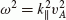is modified into the Alfvén ballooning mode relation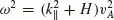(e.g., Denton, 1998; Klimushkin et al., 2004). The sign of the ballooning term H depends on the radial gradient of the plasma pressure P : for the inward gradient (westward equilibrium current in the magnetosphere) H < 0 and the system may be unstable due to ballooning instability under a sufficiently steep plasma gradient. The instability caused by hot plasma confined inside the system by the curved magnetic field tending to expand outward in a localized region.

Since the ballooning instability develops in finite-pressure inhomogeneous plasma immersed into a curved magnetic field, two factors must be taken into account: diamagnetic drift effects and the coupling between the Alfvén mode and a compressional mode (that is, the mode with a large parallel component of perturbed magnetic field). Due to the coupling, the Alfvén mode acquires a longitudinal magnetic field component (e.g., Pokhotelov et al., 1985; Klimushkin and Mager, 2011). Recently, in the single-fluid MHD treatment, Mazur et al. (2012) found that the compressional mode stabilizes the Alfvénic branch, but can be unstable itself. The influence of drift effects was studied in two-fluid MHD by Miura et al. (1989), who calculated the instability threshold of the drift Alfvén wave. A novel feature was the non-vanishing real part of the unstable mode frequency due to the influence of diamagnetic drift. However, coupling to the compressional mode was neglected. The instability threshold, under different assumptions, was also calculated in a number of other recent papers, mainly dealing with single-fluid MHD theory; to mention a few: Liu (1997), Miura (2000, 2004), Cheremnykh and Parnowski (2004), Golovchanskaya et al. (2006), Parnowski (2007), Agapitov et al. (2008).

However, the MHD approach might not be valid for ULF phenomena in finite pressure plasmas, when the parallel energy and mass flows are considered; moreover, it cannot consider the drift effects and different fractions of particles in plasma. In the more appropriate, kinetic approach, it was shown that the compressional mode frequency is proportional to the diamagnetic drift frequency ω* and does not depend on the wave vector parallel component (Pokhotelov et al., 1985; Woch et al., 1988). This mode is usually referred to as the drift compressional mode (e.g., Crabtree and Chen, 2004). The wave field structure and kinetic instabilities of the Alfvén and the drift compressional modes in the weak coupling regime were considered by Klimushkin and Mager (2011).

This paper deals with the ballooning instability in the case when the coupling between the Alfvén and drift compressional modes in a finite-β plasma immersed into a curved magnetic field are to be taken into account. As a theoretical approach, Chen and Hasegawa’s (1991) version of gyrokinetics (Catto et al., 1981; Antonsen and Lane, 1980) is used. A quasi-MHD limit is considered when the wave frequency is much larger than the magnetic drift frequency, and the wave phase velocity is much larger than the ion thermal speed.

## 2. The Model and Basic Equations

We consider a one-dimensionally non-uniform cylinder model of the magnetosphere where the magnetic field lines are concentric circles with radius R. All the plasma parameters (particle number density n(x), temperature T(x), and ambient magnetic field B(x)) vary across the magnetic shells only. Although this model is rather different from the real magnetosphere, it retains all the basic features necessary for the ballooning mode formation: magnetic field line curvature and inhomogeneous plasma pressure. The radius R has the role of the radial coordinate x. The coordinate y along the cylinder axis corresponds to the azimuthal coordinate in the magnetosphere, the coordinate l is along the magnetic field line.

Following Chen and Hasegawa (1991), we assume that the magnetospheric plasma consists of a core component which is mainly responsible for the mass density, but has a small plasma pressure, and a hot component which has a relatively small concentration, but large temperature, thus providing a high plasma pressure and large plasma to magnetic pressure ratio, β 1. The main contribution to the plasma pressure is by hot ions. The equilibrium distribution is assumed to be Maxwellian and isotropic, F = n(2πT)−3/2eε/T, where ε = υ2/2 is the particle energy per unit mass. In this case, the equilibrium condition is written as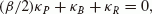(1)

where κ B = B′/B and κ P = P′/P are the normalized magnetic field and pressure gradients, κ R = R−1 is the field line curvature, prime denotes differentiation over the radial coordinate x. Note that the equilibrium current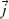is related to the pressure gradient as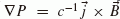, that is, κ P = jB/cP; a negative κ P value corresponds to the westward current. In the isotropic case, specific modes related to temperature anisotropy, such as the drift anisotropy mode (Pokhotelov et al., 1985) and the drift mirror mode (Woch et al., 1988; Klimushkin and Mager, 2012), are ruled out.

The dependence of the wave variables on the spatio-temporal coordinates has the form exp [−iωt + ik y y + ik l], where ω is the wave frequency, k y and k are the azimuthal and parallel components of the wave vector. The radial component of the wave vector is assumed to be zero. The ballooning approximation assumes that waves with ω k y υ A are considered, which rules out the fast magnetosonic mode.

In the gyrokinetic approach, the wave’s electromagnetic field can be described by three variables (Chen and Hasegawa, 1991). The first one (Ψ) is related to the longitudinal vector potential as follows A = −(ic/ω)∂Ψ/l. The second variable (b) is proportional to the longitudinal magnetic field of the wave b as b = ωb/c. The third variable is electrostatic potential φ. It was shown (e.g., by Chen and Hasegawa, 1991) that a small fraction of cold electrons (that is, electrons with a lower thermal speed than the parallel phase velocity of the wave) shorts out the longitudinal wave’s electric field (E = −ik (φ − Ψ) = 0); thus, the potential φ is not an independent variable, and the variables Ψ and b fully describe the electromagnetic field of the perturbation. If the electrostatic component of perturbation is considered, a great variety of wave branches and instabilities appear (Marchenko et al., 1988), but all of them become irrelevant if at least small fraction of cold electrons occurs in plasma.

The variables Ψ and b are related by the gyrokinetic equations (Antonsen and Lane, 1980; Catto et al., 1981; Chen and Hasegawa, 1991) which can be conveniently written as follows: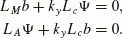(2)

Here the compressional mode operator is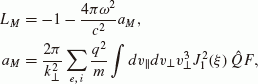(3)

whereis an argument of the Bessel function J1, ω c is gyrofrequency. The operator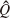is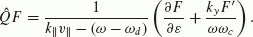Here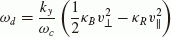is the particle drift frequency in an inhomogeneous magnetic field.

The transverse Alfvén ballooning operator is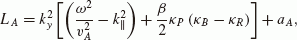(4)

where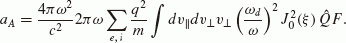Finally, the coupling operator is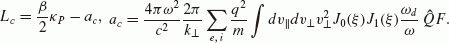The terms a M , a A , a c are responsible for wave-particle interaction. In our study, we search for hydrodynamic instability, therefore relatively weak resonant kinetic effects are neglected. In the quasi-MHD limit, the wave frequency is higher than the magnetic drift frequency, ω ω d , the wave phase velocity is much larger than the ion thermal speed, ω/k υ, and the wave perpendicular scale is much larger than the ion Larmor radius,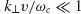. Then the Alfvén ballooning operator is recast as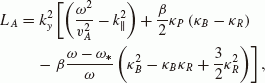(5)

where the second and third terms in brackets (proportional to β) can be called the ballooning terms. The coupling and the compressional mode operators are written as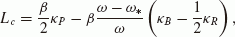(6)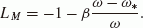(7)

Here we have introduced the plasma Larmor drift frequency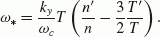(8)

Note that this value is different from the diamagnetic drift frequency obtained in the two-fluid MHD approach (see, e.g., equation (8) in Miura et al., 1989).

Expressing b from the first equation of the system (2) and substituting it into the second equation, we obtain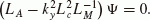(9)

It is worth noting that (as seen from Eqs. (5) and (6)) the second term in brackets (caused by the mode coupling) generally has the same order of magnitude as the ballooning additions to the Alfvén operator, which justifies the necessity of taking into account the coupling between the Alfvén and drift compressional modes. Further, Eq. (9) leads to the dispersion relation for the coupled Alfvén ballooning and drift compressional modes in a non-uniform plasma:(10)

where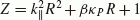and Ω M = ω*β/(1 + β) (the drift compressional frequency) is the frequency which turns the operator L M into zero.

## 3. Stability of Coupled Modes

First, let us consider the limit when the drift frequency is negligibly small, ω* → 0. Then Eq. (10) has a solution for the squared wave frequency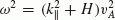with a ballooning term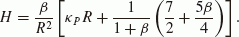(11)

When ω2< 0 the mode is unstable (Fig. 1, upper panel). The instability has an aperiodic character which means that ω2 changes its sign from positive to negative, going through zero at the instability threshold(12)

A necessary condition for the instability is a sharp decrease of plasma pressure with distance, P′ < 0. It corresponds to the westward equilibrium current.

The occurrence of a steep plasma gradient, necessary for the ballooning instability, inevitably causes the drift effects. Including the drift compressional mode and its coupling with Alfvén mode changes the situation dramatically. The dispersion relation (10) becomes a fourth-order equation with respect to ω. The real and imaginary parts of the wave frequency are depicted in Fig. 1 (lower panel).

There are four modes. The first one (labeled I in Fig. 1, lower panel) always has a real frequency. At low pressure, it corresponds to the Alfvén mode with negative frequency, ω = −kυ A . Physically, it means that the azimuthal phase speed of this wave is directed opposite to the diamagnetic drift velocity of ions. As the westward current increases, this mode frequency tends to drift compressional frequency with the minus sign, −Ω M .

The frequency of the second mode (II) changes from zero at low pressure to the drift compressional frequency for a large westward current, being always less than Ω M . Since this mode starts with the zero frequency, we are not sure whether this mode is not an artifact of the approximation assumed in this paper (ω kυ). In any case, this mode is stable.

The most interesting are the modes labeled III and IV. At low pressure, the former corresponds to the Alfvén mode with positive frequency, ω = + kυ A . The frequency decreases with the westward current. The frequency of the latter, vice versa, increases from the value Ω M . This oscillation branch is usually called the drift compressional mode. When the current is not very large, both modes have only a real frequency. Their azimuthal phase speed has the same direction as the velocity of the ion diamagnetic drift.

At some critical value j c of the current, the lines III and IV in Fig. 1 (lower panel) merge to produce a single line, V. At the same time two other lines appear in this graph, VI and VII, corresponding to mutually opposite imaginary parts of frequency. Thus, at j > j c , the four modes are I, II, VI and VII. Branches I and II have real frequencies, while branches VI and VII have complex (complex conjugate) frequencies. The situation when merging of different oscillations branches produces new, unstable modes, is typical is non-uniform plasmas (e.g., Pokhotelov et al., 1985; Klimushkin and Mager, 2012).

Thus, if the current exceeds the critical value j c , a mode appears with a complex frequency whose the real part is determined by line V, and the imaginary part (growth rate) by line VI in Fig. 1. This mode can be called the drift ballooning coupling mode.

As is seen in the lower and upper panels of Fig. 1, the instability threshold depends on Ω M . As Ω M grows, the instability occurs at lower |κ P | values. This dependence can be evaluated analytically in the limit Ω M Ω A , where Ω A = kυ A is the Alfvénic frequency in cold plasma. Then the fourth order equation (10) is reduced to a cubic equation with respect to the variable χ = ω α :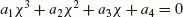with the coefficients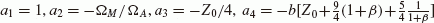. Here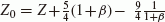. Two of three roots of this equation coincide when its discriminant is zero: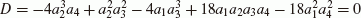(this case corresponds to the point where the branches III and IV in Fig. 1 (lower panel) merge). When D < 0, two of the three roots are complex conjugate, and one of them must be unstable. Assuming that a2 1, we found the instability threshold: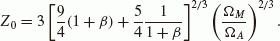(13)

Hence the critical current is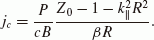Note that in the ω* → 0 limit the threshold takes the form Z0 = 0, in agreement with Eq. (12). Thus, the higher the diamagnetic frequency ω*, the lower the westward current where the instability takes place.

## 4. Discussion

Let us compare our results with those in previous works. In the low-pressure limit (0 < β 1) the ballooning term (11) takes the form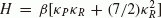, which coincides with the one obtained in papers dealing with MHD theory (e.g., low-β limit of Denton, 1998; Klimushkin et al., 2004; Mazur et al., 2012) at the adiabatic index γ = 7/4. There is a difference (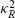terms) with the ballooning term obtained in Miura et al. (1989) and Miura (2000, 2004).

A consequence of difference between the ballooning terms obtained in different papers is a difference between the ballooning instability thresholds found in different works (e.g., Liu, 1997; Mazur et al., 2012 versus Miura et al., 1989; Miura, 2000, 2004). This difference must be associated with different assumptions regarding the splitting of the whole wave field into the Alfvén and compressional parts and should be clarified in further studies, mainly in MHD theory (see, however, some discussion of the issue in Mazur et al., 2012). In our case, we do not perform this splitting at all: the instability occurs when the Alfvén and compressional branches merge. The ballooning instability threshold obtained in the ω* → 0 limit (12) is different from the threshold obtained by Miura et al. (1989), but practically coincides (neglecting the third term) with the one obtained by Liu (1997) and Mazur et al. (2012). One cannot be completely sure, however, that this coincidence is not by chance, since those papers used completely different models of medium (gyrokinetic in our case, MHD in theirs) which leads to a different dispersion relation for the compressional mode.

An important result of this study is that introducing coupling between the Alfvén mode and the drift compressional mode (with the characteristic frequency Ω M ) produces a real part of the frequency of the ballooning-unstable mode. It is necessary to note that a similar feature has already been found by Miura et al. (1989) in their study of the drift Alfvén mode stability in terms of two-fluid MHD theory. However, their basic assumptions are very different from those employed in our paper. Miura et al. (1989) considered the drift Alfvén mode using the dispersion relation modified according to the rule ω2ω(ωω*) (e.g., Krall and Trivelpiece, 1973). Vice versa, this kind of the Alfvén mode modification was neglected in our paper, since we assumed the fraction of hot particles to be much less abundant than the fraction of cold particles, n h /n c 1. For this reason the diamagnetic term in the Alfvénic dispersion relation is n h /n c times reduced (e.g., Pokhotelov et al., 1985) and can be neglected. Then, we took into account the coupling between the Alfvén and compressional modes, neglected by Miura et al. (1989). However, this mode had contributions to the final dispersion relation of the same order as those came from the Alfvén mode itself. It is the (drift) compressional mode which brought forth the diamagnetic drift frequency in our study. Respectively, the expressions for this frequency in this study are quite different from those in Miura et al. (1989). Therefore, although the outcome—appearance of a real part of frequency of the ballooning-unstable mode—is the same in these two studies, it is caused by completely different physical factors.

## 5. Conclusion

Below the instability threshold, a general dispersion relation for the ballooning modes is found to be comprised of two branches, the Alfvén and the drift compressional ones. As the westward current grows, these two branches become more and more close to each other merging at some critical value of the current. This critical value depends on the drift to Alfvénic frequency ratio, field line curvature, and plasma beta. Then, the single mode appears, which is called the drift ballooning coupling mode. The unstable mode has the same direction of the azimuthal propagation as the velocity of ion diamagnetic drift (ω M > 0).

This mode has both the real and imaginary parts of the frequency, the latter corresponding to the instability. The criterion of the instability has been obtained. It was found that it depends on the drift frequency: As Ω M decreases, the real part of the frequency tends to zero, thus the behavior of the drift ballooning coupling instability resembles the behavior of the ordinary MHD ballooning instability. The higher the diamagnetic frequency ω*, the lower the westward current needed for the instability.

Although different in detail from the results of previous works (differing from each other as well), our paper confirms the finding that the instability develops under a strong westward current. The finding that the diamagnetic drift effects cause the real part of the unstable mode frequency confirms an earlier result by Miura et al. (1989), although for different physical reasons.

## References

• Agapitov, A. V., O. K. Cheremnykh, and A. S. Parnowski, Ballooning perturbations in the inner magnetosphere of the Earth: Spectrum, stability and eigenmode analysis, Adv. Space Res., 41, 1682–1687, 2008.

• Antonsen, T. M. and B. Lane, Kinetic equations for low frequency instabilities in inhomogeneous plasma, Phys. Pluids, 23, 1205–1214, 1980.

• Catto, P. J., W. M. Tang, and D. E Baldwin, Generalized gyrokinetics, Plasma Phys., 23, 639–650, 1981.

• Chen, L. and A. Hasegawa, Kinetic theory of geomagnetic pulsations, 1, Internal excitations by energetic particles, J. Geophys. Res., 96, 1503–1512, 1991.

• Cheng, C. Z., Physics of substorm growth phase, onset, and dipolarization, Space Sci. Rev., 113, 207–270, 2004.

• Cheremnykh, O. K. and A. S. Parnowski, The theory of ballooning perturbations in the inner magnetosphere of the Earth, Adv. Space Res., 33, 769–773, 2004.

• Crabtree, C. and L. Chen, Finite gyroradius theory of drift compressional modes, Geophys. Res. Lett., 31, L17804, doi:10.1029/2004GL020660, 2004.

• Denton, R. E., Compressibility of the poloidal mode, J. Geophys. Res., 103, 4755–4760, 1998.

• Golovchanskaya, I. V., A. Kullen, Y. P. Maltsev, and H. Biernat, Ballooning instability at the plasma sheet-lobe interface and its implications for polar arc formation, J. Geophys. Res., 111, A11216, doi:10.1029/2005JA011092, 2006.

• Klimushkin, D. Yu. and P. N. Mager, Spatial structure and stability of coupled Alfvén and drift compressional modes in non-uniform magnetosphere: gyrokinetic treatment, Planet. Space Sci., 59, 1613–1620, doi: 10.1016/j.pss.2011.07.010, 2011.

• Klimushkin, D. Yu. and P. N. Mager, Coupled Alfvén and drift-mirror modes in non-uniform space plasmas: a gyrokinetic treatment, Plasma Phys. Control. Fusion, 54, 015006 (10pp), doi:10.1088/0741-3335/54/1/015006, 2012.

• Klimushkin, D. Yu., P. N. Mager, and K.-H. Glassmeier, Toroidal and poloidal Alfvén waves with arbitrary azimuthal wave numbers in a finite pressure plasma in the Earth’s magnetosphere, Ann. Geophys., 22, 267–288, 2004.

• Krall, N. A. and A. W. Trivelpiece, Principles of Plasma Physics, McGraw-Hill Book Company, 1973.

• Liu, W. W., Physics of the explosive growth phase: Ballooning instability revisited, J. Geophys. Res., 102, 4927–4931, 1997.

• Marchenko, V. A., Yu. M. Nezlina, and O. A. Pokhotelov, Drift field-swelling instability in anisotropic plasmas, Plasma Phys. Control. Fusion, 30, 957–966, 1988.

• Mazur, N. G., E. N. Fedorov, and V. A. Pilipenko, Dispersion relation for ballooning modes and condition of their stability in the near-Earth plasma, Geomagn. Aeron., 52, 603–612, 2012.

• Miura, A., Conditions for the validity of the incompressible assumption for the ballooning instability in the long-thin magnetospheric equilibrium, J. Geophys. Res., 105, 18793–18806, 2000.

• Miura, A., Balooning instability as a mechanism of the near-Earth onset of substorms, Space Sci. Rev., 195, 387–398, 2001.

• Miura, A., Validity of the fluid description of critical β and Alfvén time scale of ballooning instability onset in the near-Earth collisionless high-β plasma, J. Geophys. Res., 109, A02211, doi:10.1029/2003JA009924, 2004.

• Miura, A., S. Ohtani, and T. Tamao, Ballooning instability and structure of diamagnetic hydromagnetic waves in a model magnetosphere, J. Geophys. Res., 94, 15231–15242, 1989.

• Ohtani, S. and T. Tamao, Does the ballooning instability trigger substorms in the near-Earth magnetotail?, J. Geophys. Res., 98, 19369–19379, 1993.

• Parnowski, A. S., Eigenmode analysis of ballooning perturbations in the inner magnetosphere of the Earth, Ann. Geophys., 25, 1391–1403, 2007.

• Pokhotelov, O. A., V. A. Pilipenko, and E. Amata, Drift anisotropy instability of a finite-β magnetospheric plasma, Planet. Space Sci., 33, 1229–1241, 1985.

• Woch, J., G. Kremser, O. A. Pokhotelov, V. A. Pilipenko, and E. Amata, Curvature-driven drift mirror instability in the magnetosphere, Planet. Space Sci., 36, 383–393, 1988.

## Acknowledgements

The work was supported by RFBR grants 12-05-00121-a and 12-05-98522-vostok-a, Program of presidium of Russian Academy of Sciences #22.

## Author information

Authors

### Corresponding author

Correspondence to D. Yu. Klimushkin.

## Rights and permissions

The images or other third party material in this article are included in the article’s Creative Commons licence, unless indicated otherwise in a credit line to the material. If material is not included in the article’s Creative Commons licence and your intended use is not permitted by statutory regulation or exceeds the permitted use, you will need to obtain permission directly from the copyright holder.

To view a copy of this licence, visit https://creativecommons.org/licenses/by/4.0/.

Reprints and Permissions

Klimushkin, D.Y., Mager, P.N. & Pilipenko, V.A. On the ballooning instability of the coupled Alfvén and drift compressional modes. Earth Planet Sp 64, 777–781 (2012). https://doi.org/10.5047/eps.2012.04.002

• Revised:

• Accepted:

• Published:

• Issue Date:

• DOI: https://doi.org/10.5047/eps.2012.04.002

### Key words